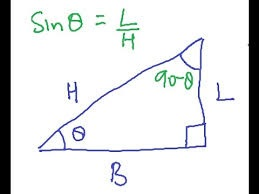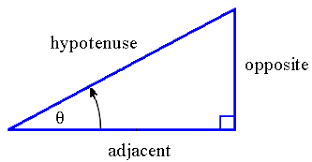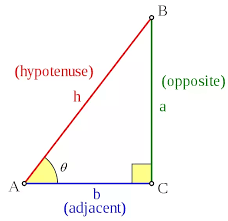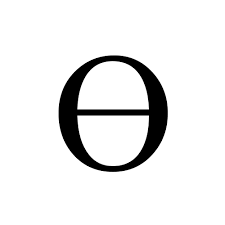# What is Theta:

General Questions, those who prepare for competitive exams.
This is basic information to understand at a glance.

What is Theta ϴ: The measure of an angle is Theta.
What will have Triangle: Base, Length, Hypotenus.H: Hypotenuse.
L: Length
B: Base

What is Sin ϴ (Sin Theta): Sin ϴ = L/H

If L & H are same Length then symbol we can write like this:

If L is 0 Hypotenuse is equivalent to 0. Then Sin Theta is equivalent to 0. Sin ϴ = 0

If Base is 0 L=H. So sin Sin ϴ (90 degrees ) = 1.

Sin of 180 degrees = sign theta of zero = 0.

Sin ϴ = (270 degrees ) = -1. Because H = -L

Sin (360) = 0.Sin ϴ = Length / Hypotenuse ( L/H).
Cos ϴ = Base /Hypotenuse (B/H).
Tan ϴ = Length/Base (L/B).

Wishing you all the best,
http://www.seeyourneeds.in DONATE AS YOU LIKE